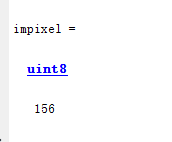• matlab获取图像像素值
千次阅读
2021-04-18 03:49:04

我在MATLAB中有一个图像：

我想做一些处理：

pic = some_processing(y);

并找到输出的本地最大值。也就是说，y中的所有要点都大于所有邻居。

我似乎找不到一个很好的MATLAB函数。我能想出的最好的是：

[dim_y,dim_x]=size(pic);

enlarged_pic=[zeros(1,dim_x+2);

zeros(dim_y,1),pic,zeros(dim_y,1);

zeros(1,dim_x+2)];

% now build a 3D array

% each plane will be the enlarged picture

% moved up,down,left or right,

% to all the diagonals, or not at all

[en_dim_y,en_dim_x]=size(enlarged_pic);

three_d(:,:,1)=enlarged_pic;

three_d(:,:,2)=[enlarged_pic(2:end,:);zeros(1,en_dim_x)];

three_d(:,:,3)=[zeros(1,en_dim_x);enlarged_pic(1:end-1,:)];

three_d(:,:,4)=[zeros(en_dim_y,1),enlarged_pic(:,1:end-1)];

three_d(:,:,5)=[enlarged_pic(:,2:end),zeros(en_dim_y,1)];

three_d(:,:,6)=[pic,zeros(dim_y,2);zeros(2,en_dim_x)];

three_d(:,:,7)=[zeros(2,en_dim_x);pic,zeros(dim_y,2)];

three_d(:,:,8)=[zeros(dim_y,2),pic;zeros(2,en_dim_x)];

three_d(:,:,9)=[zeros(2,en_dim_x);zeros(dim_y,2),pic];

然后看看第三层的最大值是否出现在第一层(即：three_d(：，：，1))中：

(max_val, max_i) = max(three_d, 3);

result = find(max_i == 1);

有没有更优雅的方式来做到这一点？这似乎是一个肮脏的一点。

更多相关内容
• 获取任意图像位置的像素值 根据坐标获取像素值 P=impixel(im,r,c) r:行坐标 c:纵坐标 P：返回的RGB值 im=imread('C:\Users\14372\Desktop\Music_pictures\图片\梦.png'); r=[40 60 80]; c=[100 120 140]; figure; ...
• 获取任意图像位置的像素值

根据坐标获取像素值

P=impixel(im,r,c)

r:行坐标 c:列坐标

P：返回的RGB值

im=imread('C:\Users\14372\Desktop\Music_pictures\图片\梦.png');
r=[40 60 80];
c=[100 120 140];
figure;
imshow(im);
p=impixel(im,r,c)

%结果
每一行表示一个像素点的rgb值
p =

15    19    30
12    14    26
13    19    31


根据鼠标获取像素值

im=imread('C:\Users\14372\Desktop\Music_pictures\图片\梦.png');
figure;
imshow(im);
h=impixelinfo;%impixelinfo能够在当前绘图窗口（figure）中显示绘制图像的像素信息
set(h,'position',[10 10 200 50]);%显示框• 制作动图
展开全文1、matlab获取彩色图像像素值的方法

img1=img(:,:,1);

img2=img(:,:,2);

img3=img(:,:,3);

三通道排序分别对应彩色图像的rgb三通道

2、opencv中获取图像像素的方法

方法一：

CvScalar pixel;

for (int i = 0; i < img->height; ++i)

{

for (int j = 0; j < img->width; ++j)

{

//获得像素的RGB值并显示

pixel = cvGet2D(img, i, j);

printf("B=%f,G=%f,R=%f\t", pixel.val, pixel.val, pixel.val);

//修改各点的值

pixel.val = 0;   // B分量

pixel.val = 0;   // G分量

pixel.val = 0;   // R分量

cvSet2D(img, i, j, pixel);

}

}

注意内存中存储顺序是BGR

方法二：

for (int i = 0; i < img->height; ++i)

{

for (int j = 0; j < img->width; ++j)

{

int  b = CV_IMAGE_ELEM(paintx, uchar, y1, x1 * 3);

int  g = CV_IMAGE_ELEM(paintx, uchar, y1, x1 * 3+1);

int  r= CV_IMAGE_ELEM(paintx, uchar, y1, x1 * 3+2);

}

}

方法三：

对于单通道字节型图像：

IplImage* img=cvCreateImage(cvSize(640,480),IPL_DEPTH_8U,1);

((uchar *)(img->imageData + i*img->widthStep))[j]=111;

对于多通道字节型图像：

IplImage* img=cvCreateImage(cvSize(640,480),IPL_DEPTH_8U,3);

((uchar *)(img->imageData + i*img->widthStep))[j*img->nChannels +0]=111;//B

((uchar *)(img->imageData + i*img->widthStep))[j*img->nChannels +1]=112;//G

((uchar *)(img->imageData + i*img->widthStep))[j*img->nChannels +2]=113;//R

对于多通道浮点型图像：

IplImage* img=cvCreateImage(cvSize(640,480),IPL_DEPTH_32F,3);

((float*)(img->imageData + i*img->widthStep))[j*img->nChannels +0]=111;//B

((float*)(img->imageData + i*img->widthStep))[j*img->nChannels +1]=112;//G

((float*)(img->imageData + i*img->widthStep))[j*img->nChannels +2]=113;//R

3 ((uchar*)(Img1->imageData + Img1->widthStep*pt.y))[pt.x]的具体含义：

(1)这里img1->imagedata指的图像第一行的首地址，pt.y是指图像的height(图像高像素数即行数),y乘以img->widthstep(排列的图像行大小)即首地址加上行数乘以每行总数，得到第y行的首地址。widthstep是指图像每行所占的字节数，主要要和width区别，width是指每行所含的像素个数，但是一个像素也可能占一个字节，也可能占三个字节或者四个。imagedata是指向存储图像像素值数组的指针，内容是这个数组的首地址，pt.y指的是像素点的行坐标，所以Img->imageData + Img->widthStep*pt.y便是该像素点所在行的首地址，然后再加上该像素点所在的列，即pt.x，就得到了该像素点的地址，所以那句代码也可以写成

((uchar*)(Img1->imageData + Img1->widthStep*pt.y+pt.x))，

都是指该像素点的像素值，至于强制类型转换，是因为会产生一些负值，而像素值是不能为负的。

(2)widthStep表示存储一行像素需要的字节数。

widthStep必须是4的倍数，如果8U图像宽度为3，那么widthStep是4，加一个字节补齐。这个图像的一行需要4个字节，只使用前3个，最后一个空在那儿不用。也就是一个宽3高3的图像的imageData数据大小为4*3=12字节。如果32F的图像，那么widthStep=width*4。

参考：

展开全文• Matlab如何用鼠标获取图像像素值和坐标-test.m 如题 我是在一个GUI里，有两个axe，分别用来读取两个图片 我想要实现的是：图片在axe显示后，当鼠标在图片上移动，或者点击，可以得到图片的像素值和其在axe中的...
• 基于matlab读取图像文件并，并计算其图像对比度。 计算公式采用：各中心像素灰度与周围8近邻像素灰度之差的平方之和再除以差的个数。 注：直接运行，选取路径即可输出计算结果，十分方便。适用于大量图片待...
• Matlab如何用鼠标获取图像像素值和坐标-test.fig 如题 我是在一个GUI里，有两个axe，分别用来读取两个图片 我想要实现的是：图片在axe显示后，当鼠标在图片上移动，或者点击，可以得到图片的像素值和其在axe中...
• 灰度线性变换是最常⽤的图像点运算之⼀，将图像像素值通过指定的线性函数进⾏变换，以此增强或减弱图像的灰度。 程序主要实现以下功能 1) 显示原始图像，并求其原始图像的直方图 2) 通过⼀维线性函数增强对比度，...
• 这包含一个 Matlab 程序，您可以在其中将像素值提取到文本文件和另一个程序以从文本文件中提取 rgb 值并重新生成图像
• MATLAB怎么获取任意像素点的灰度 这两天跑代码遇到一个问题，想要根据图像的灰度进行阈值分割，通过学习，总结以下两种方法来对任意像素点的灰度。 方法一 1.首先取得你想要的点的坐标，通过ginput函数，...

## 用MATLAB怎么获取任意像素点的灰度值

这两天跑代码遇到一个问题，想要根据图像的灰度值进行阈值分割，通过学习，总结以下两种方法来对任意像素点的灰度值。
方法一
1.首先取得你想要的点的坐标，通过ginput函数，然后在出现的图像上“点击你想要的点”，然后“回车”

I = rgb2gray(I); %转化为单通道灰度图

[y0,x0]=ginput()
y0=round(y0); %round代表四舍五入
x0=round(x0);


2.通过impixel函数获取该点的灰度值

impixel = I(x0,y0)


具体示例代码：

I=imread('你的图片.JPG');
I = rgb2gray(I);
imshow(I);
[y0,x0]=ginput();
y0=round(y0);
x0=round(x0);
impixel = I(x0,y0)


结果：方法二
使用matlab自带工具“数据游标”就可以显示，如下所示：点击你想要的点就可以显示：当时RGB图像时，没有经过灰度处理，所显示的就是对应的R、G、B的值，当灰度化后，可看到RGB相等，得到的就是灰度值。展开全文开发语言 python
• 输入 clear all； RGB = imread（‘图片名.格式’）； r = [x1 y1 z1];%指定像素点的横坐标 ...p = impixel(RGB,r,c）%用impixel（）函数来返回RGB图像的横纵坐标对应的像素点的 输出： p = a b c d e f g h i ...
• ## matlab获取图像像素坐标[已验证]

万次阅读 多人点赞 2018-05-23 09:29:01
1. impixelinfo%例如: %1.1 显示图片 mainf=imshow(data); %show the picture %1.2 命令框中... ginput[x,y] = ginput(n)%例如: %显示图片 mainf=imshow(data); %show the picture%↓返回的x ↓返回的x ...
• 统计二化之后，各个黑白图像中0、1 的像素点数目 使用折线图的方式，展示出统计的结果 首先进行输入文件夹 与输出目标文件夹的路径定义： input_path = 'E:\test1\';%输入图片文件夹路径 output_path = 'E:\...
• 当我们读取已经保存好的图片（jpg BMP等格式）时，用image imshow等函数显示经常会有失真效果，按照以下步骤可以使得显示的图片效果更清晰： set(0,'units','pixes'); %获取图片的屏幕的分辨率 screensize0 = get...
• 1. 先将那个图片显示出来 img_a = imread('test.tiff'); %写文件名字，文件放在matlab可检索的目录下 imshow(img_a); 2. 点击左上角的标识符号 ...
• 利用Matlab读取图片各个像素的RGB颜色，然后在Excel中将各像素RGB颜色对应地填充到单元格中。
• ## MATLAB图像处理之图像的像素矩阵

万次阅读 多人点赞 2018-10-02 17:47:40
首先我们用MATLAB打开一张图像可以发现在工作区中就会多一个变量，这个变量是一个三维数组，第一维代表图像的X坐标，第二维代表图像的Y坐标，第三维代表R、G、B，第三维中的数字1代表R，数字2代表G，数字3代表B。...
• 点运算又称为对比度增强、对比度拉伸或灰度变换，是一种通过图像中的每一个像素值(即像素点上的灰度值)进行运算的图像处理方式。它将输入图像映射为输出图像，输出图像每个像素点的灰度值仅由对应的输入像素点的灰度...
• 基于MATLAB的数字图像处理环境，介绍了如何利用MATLAB及其图像处理工具箱进行数字图像处理。主要论述了利用MATLAB的GUI实现直方图均衡化、阀化、加噪、锐化以及存储等图像处理。计算机视觉
• %生成头骨幻影图像 imshow§%显示头骨幻影图像 theta1 = 0:10:170;%投影的角度，步长为10 [R1,xp] = radon(P,theta1);%radon变换 num_angles_R1 = size(R1,2);%角度的个数 theta2 = 0:5:175;%投影角度，步长为5 [R2,...计算机视觉 opencv
• 读取一幅图像图像奇数位置（13579…）的像素值每个点的像素值增加50图像偶数位置（2468….）的像素值每个点的像素值减小50在同一个绘图窗口分别显示原始图像和处理后的图像
• ## matlab像素值及统计

千次阅读 2021-04-18 08:00:00
1.像素数据impixel函数可以返回用户指定的图像像素的颜色数据，impixel函数可以返回选中像素或者是像素集的数据，可以直接将像素坐标作为该函数的输入参数，或用鼠标选中像素。imshow trees.tifvals=impixel...
• 灰度数字图像是每个像素只有一个采样颜色的图像。这类图像通常显示为从最暗黑色到最亮的白色的灰度，尽管理论上这个采样可以任何颜色的不同深浅，甚至可以是不同亮度上的不同颜色。灰度图像与黑白图像不同，在计算机...
• ## python读取像素值

千次阅读 2022-01-11 19:17:36python 计算机视觉
• MATLAB图片点的像素坐标，可以精准的查看图片上每一个点的坐标，很精确，达到0.0001个像素
• 获取图像内向量的所有像素坐标 我有一个强度/灰度图像，并且在该图像中选择了一个像素。我想从所有方向/角度从该像素开始发送矢量，并且我想对所有矢量求和触摸一个矢量的像素的所有强度的总和。在此步骤之后，我想...
• 我正在使用Kinect v2，我必须将深度信息映射到RGB图像上来处理它们：特别是，我需要知道RGB图像中哪些像素在Z的某个距离(深度)范围内轴;我正在使用C＃程序获取所有数据并将其保存为图像(RGB)和txt文件(深度) .我遵循......

# matlab获取图像像素值matlab 订阅